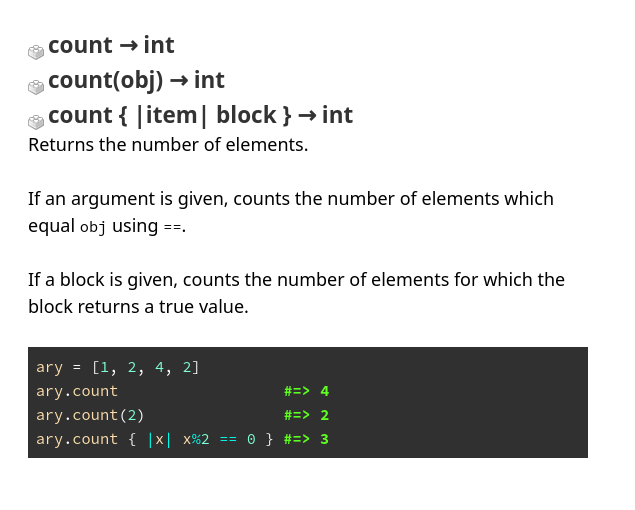Useful resources when studying Ruby's internals

I'm gonna list a few places I visit whenever I want to learn more about a specific part of Ruby.

Ruby MRI on Github

The whole source code is mirrored there. Here you can learn how the language is implemented.

For example, if we want to learn about the Array class, we can look in array.c.

Let's say I'm interested in the Array#count method:

static VALUE
rb_ary_count(int argc, VALUE *argv, VALUE ary)
{
long i, n = 0;

if (rb_check_arity(argc, 0, 1) == 0) {
VALUE v;

if (!rb_block_given_p())
return LONG2NUM(RARRAY_LEN(ary));

for (i = 0; i < RARRAY_LEN(ary); i++) {
v = RARRAY_AREF(ary, i);
if (RTEST(rb_yield(v))) n++;
}
}
else {
VALUE obj = argv;

if (rb_block_given_p()) {
rb_warn("given block not used");
}
for (i = 0; i < RARRAY_LEN(ary); i++) {
if (rb_equal(RARRAY_AREF(ary, i), obj)) n++;
}
}

return LONG2NUM(n);
}

// ...

rb_define_method(rb_cArray, "count", rb_ary_count, -1);

The Ruby Spec Suite

Let's say I'm interested in the Array#count method:

describe "Array#count" do
it "returns the number of elements" do
[:a, :b, :c].count.should == 3
end

it "returns the number of elements that equal the argument" do
[:a, :b, :b, :c].count(:b).should == 2
end

it "returns the number of element for which the block evaluates to true" do
[:a, :b, :c].count { |s| s != :b }.should == 2
end
end

Ruby-doc

Documentation for pretty much every versions of Ruby MRI.

Here is the doc for Array#countThe Rubinius implementation

Rubinius is basically Ruby implemented in Ruby. It's fun to compare with the original C implementation.

For example, here is the source code for Array#count:

def count(item = undefined)
seq = 0
if !undefined.equal?(item)
each do
element = Rubinius.single_block_arg
seq += 1 if item == element
end
elsif block_given?
each { |element| seq += 1 if yield(element) }
else
each { seq += 1 }
end
seq
end

Does it look like it match the Ruby spec?

JRuby, Ruby on the JVM

I don't know Java but this project motivate me to learn about it.

Here is Array#count source code from JRuby's RubyArray.java:

@JRubyMethod(name = "count")
public IRubyObject count(ThreadContext context, Block block) {
if (block.isGiven()) {
int n = 0;
for (int i = 0; i < realLength; i++) {
if (block.yield(context, elt(i)).isTrue()) n++;
}
return RubyFixnum.newFixnum(context.runtime, n);
} else {
return RubyFixnum.newFixnum(context.runtime, realLength);
}
}

@JRubyMethod(name = "count")
public IRubyObject count(ThreadContext context, IRubyObject obj, Block block) {
if (block.isGiven()) context.runtime.getWarnings().warn(ID.BLOCK_UNUSED, "given block not used");

int n = 0;
for (int i = 0; i < realLength; i++) {
if (equalInternal(context, elt(i), obj)) n++;
}
return RubyFixnum.newFixnum(context.runtime, n);
}

Those are methods of the Java class RubyArray.

Bonus, the Mailing Lists Archive for Ruby MRI
Summary

Regarding gems and their documentation (offtopic but cool to have anyway):

Anything to add to the list?

Written by Tanguy Andreani
on 19th of July